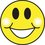# Help Me! Functions, limits and continuity

Hey guys,

Today I came across a question about the function $f(x)$, which takes the value $x$ when $x \in Q$ and the value $1-x$ when $x \notin Q$. Is this function continuous at any point?

I thought that $f(x)$ would be continuous at $x=0.5$ since the left and right hand limits seem to approach $0.5$ near $x=0.5$.

Am I correct? If so, why? If not, why?

Thanks!Note by Raj Magesh
6 years ago

This discussion board is a place to discuss our Daily Challenges and the math and science related to those challenges. Explanations are more than just a solution — they should explain the steps and thinking strategies that you used to obtain the solution. Comments should further the discussion of math and science.

When posting on Brilliant:

• Use the emojis to react to an explanation, whether you're congratulating a job well done , or just really confused .
• Ask specific questions about the challenge or the steps in somebody's explanation. Well-posed questions can add a lot to the discussion, but posting "I don't understand!" doesn't help anyone.
• Try to contribute something new to the discussion, whether it is an extension, generalization or other idea related to the challenge.
• Stay on topic — we're all here to learn more about math and science, not to hear about your favorite get-rich-quick scheme or current world events.

MarkdownAppears as
*italics* or _italics_ italics
**bold** or __bold__ bold
- bulleted- list
• bulleted
• list
1. numbered2. list
1. numbered
2. list
Note: you must add a full line of space before and after lists for them to show up correctly
paragraph 1paragraph 2

paragraph 1

paragraph 2

[example link](https://brilliant.org)example link
> This is a quote
This is a quote
    # I indented these lines
# 4 spaces, and now they show
# up as a code block.

print "hello world"
# I indented these lines
# 4 spaces, and now they show
# up as a code block.

print "hello world"
MathAppears as
Remember to wrap math in $$ ... $$ or $ ... $ to ensure proper formatting.
2 \times 3 $2 \times 3$
2^{34} $2^{34}$
a_{i-1} $a_{i-1}$
\frac{2}{3} $\frac{2}{3}$
\sqrt{2} $\sqrt{2}$
\sum_{i=1}^3 $\sum_{i=1}^3$
\sin \theta $\sin \theta$
\boxed{123} $\boxed{123}$

## Comments

Sort by:

Top Newest

Do you know what is the delta epsilon definition of a limit?

Staff - 6 years ago

Log in to reply

Thanks a lot! I went through the wiki and worked out the answer to my question. The epsilon-delta definition is broader than the one I learnt in school...

- 5 years, 12 months ago

Log in to reply

hi

- 5 years, 6 months ago

Log in to reply

Hey.

- 5 years, 6 months ago

Log in to reply

How are you

- 5 years, 6 months ago

Log in to reply

Great, you?

- 5 years, 6 months ago

Log in to reply

These are called drichlet functions and these are continuos when both equations are equal

- 6 years ago

Log in to reply

×

Problem Loading...

Note Loading...

Set Loading...